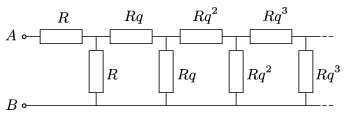Mathematical and Physical Journal
for High Schools
Issued by the MATFUND Foundation
 Already signed up? New to KöMaL?

#Problem P. 4577. (November 2013)

P. 4577. What is the equivalent resistance of the infinite resistor chain, shown in the figure, between the points A and B? Plot the equivalent resistance versus q graph.(5 pont)

Deadline expired on December 10, 2013.

Sorry, the solution is available only in Hungarian. Google translation

Megoldásvázlat. $\displaystyle R_\text{eredő}=\left( 1-\frac{1}{2q}+ \sqrt{1+\frac{1}{4q^2}}\,\right)R.$

### Statistics:

 33 students sent a solution. 5 points: Antalicz Balázs, Balogh Menyhért, Bereczki Zoltán, Csathó Botond, Csibi Levente, Fehér Zsombor, Fekete Panna, Holczer András, Horicsányi Attila, Janzer Barnabás, Kasza Bence, Németh Gergely, Sal Kristóf, Szirbik Bence, Tatár Dániel, Trócsányi Péter, Zarándy Álmos. 4 points: Iván Balázs, Juhász Péter, Kacz Dániel, Seress Dániel, Szathmári Balázs. 3 points: 3 students. 2 points: 3 students. 1 point: 4 students. 0 point: 1 student.

Problems in Physics of KöMaL, November 2013